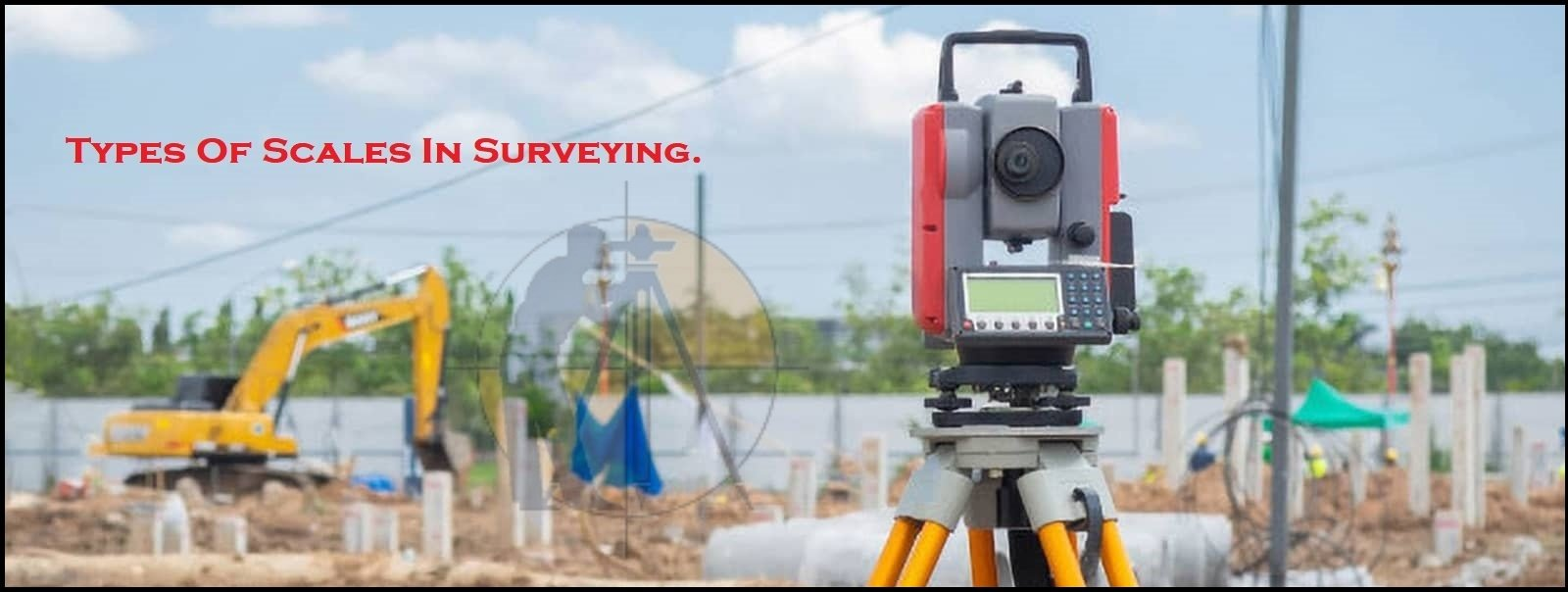Breaking News
Home / Land Surveying / Types Of Scales In Surveying

# Types Of Scales In Surveying

## Types Of Scales In The Surveying.

Since the Surveyed area is the very large, it is never possible to make full size of drawing. For convenience it is generally necessary to draw them to the reduced size, this operation is called ( drawing to scale).There are 2 types of scales used in Surveying.

1 –  Numerical scale.

2 – Graphical scale.

### A – Numerical Scale.

Numerical Scale is also further classified into 2 general types.

1 – Engineer’s Scale.

2 – Fraction Scale.

### 1 – Engineer’s Scale.

In an Engineer’s scale, the scale of the map or drawing is the proportion which every distance on the map or drawing bears to corresponding distance on the ground.

1. The Role and Responsibilities of QTY Surveyor in the Current Field Of Construction Sector

3. ### Why Camber Provided In Road

Example: One cm = Ten m

This means, One cm on the drawing represents Ten m on the ground, the scale of the map is 10 m to One cm.

### 2 – Fraction Scale.

The scale is also expressed by means of the vulgar fraction whose numerator is invariable unity. The fraction is called (Representative Fraction) or (RF). It is therefore, the ratio of the map distance to the corresponding ground distance. In forming the representative fraction both numerator & denominator must be reduced to same denomination.

For example, if the scale is Ten m to 1 cm, the RF of the scale is One cm/10x100cm = 1/1000

### B – Graphical Scale.

On the graphical scale, the units of the measurement should always be stated.

Characteristics Of the Good Scale are.

1.  It should read to the greatest accuracy required.
2.  It should be the convenient to use.

## . Land Surveying & Architects

### THANKS.